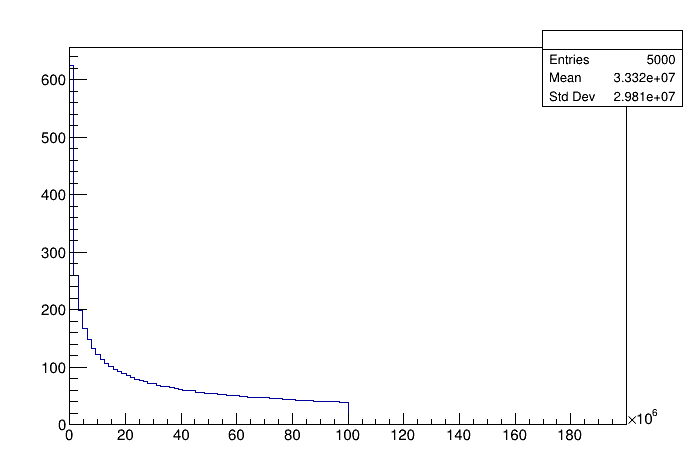# Df 0 0 7_Snapshot¶

Write ROOT data with RDataFrame.

This tutorial shows how to write out datasets in ROOT format using the RDataFrame

Author: Danilo Piparo (CERN)
This notebook tutorial was automatically generated with ROOTBOOK-izer from the macro found in the ROOT repository on Thursday, June 24, 2021 at 07:11 AM.

A simple helper function to fill a test tree: this makes the example stand-alone.

In :
%%cpp -d
void fill_tree(const char *treeName, const char *fileName)
{
ROOT::RDataFrame d(10000);
int i(0);
d.Define("b1", [&i]() { return i; })
.Define("b2",
[&i]() {
float j = i * i;
++i;
return j;
})
.Snapshot(treeName, fileName);
}


We prepare an input tree to run on

In :
auto fileName = "df007_snapshot.root";
auto outFileName = "df007_snapshot_output.root";
auto outFileNameAllColumns = "df007_snapshot_output_allColumns.root";
auto treeName = "myTree";
fill_tree(treeName, fileName);


We read the tree from the file and create a rdataframe.

In :
ROOT::RDataFrame d(treeName, fileName);


## Select entries¶

We now select some entries in the dataset

In :
auto d_cut = d.Filter("b1 % 2 == 0");


## Enrich the dataset¶

Build some temporary columns: we'll write them out

In :
auto d2 = d_cut.Define("b1_square", "b1 * b1")
.Define("b2_vector",
[](float b2) {
std::vector<float> v;
for (int i = 0; i < 3; i++)
v.push_back(b2 * i);
return v;
},
{"b2"});


## Write it to disk in ROOT format¶

We now write to disk a new dataset with one of the variables originally present in the tree and the new variables. The user can explicitly specify the types of the columns as template arguments of the Snapshot method, otherwise they will be automatically inferred.

In :
d2.Snapshot(treeName, outFileName, {"b1", "b1_square", "b2_vector"});


Open the new file and list the columns of the tree

In :
TFile f1(outFileName);
auto t = f1.Get<TTree>(treeName);
std::cout << "These are the columns b1, b1_square and b2_vector:" << std::endl;
for (auto branch : *t->GetListOfBranches()) {
std::cout << "Branch: " << branch->GetName() << std::endl;
}
f1.Close();

These are the columns b1, b1_square and b2_vector:
Branch: b1
Branch: b1_square
Branch: b2_vector


We are not forced to write the full set of column names. we can also specify a regular expression for that. In case nothing is specified, all columns are persistified.

In :
d2.Snapshot(treeName, outFileNameAllColumns);


Open the new file and list the columns of the tree

In :
TFile f2(outFileNameAllColumns);
t = f2.Get<TTree>(treeName);
std::cout << "These are all the columns available to this dataframe:" << std::endl;
for (auto branch : *t->GetListOfBranches()) {
std::cout << "Branch: " << branch->GetName() << std::endl;
}
f2.Close();

These are all the columns available to this dataframe:
Branch: b1_square
Branch: b2_vector
Branch: b1
Branch: b2


We can also get a fresh rdataframe out of the snapshot and restart the analysis chain from it. The default columns are the one selected. Notice also how we can decide to be more explicit with the types of the columns.

In :
auto snapshot_df = d2.Snapshot<int>(treeName, outFileName, {"b1_square"});
auto h = snapshot_df->Histo1D();
auto c = new TCanvas();
h->DrawClone();

return 0;


Draw all canvases

In :
gROOT->GetListOfCanvases()->Draw()